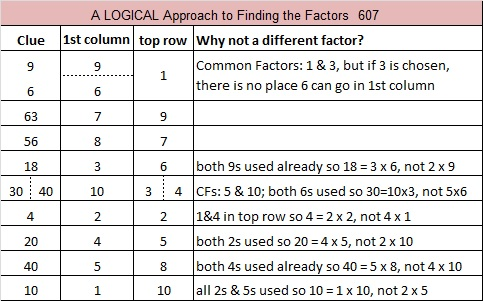# 607 and Level 6

607 is the sum of the three prime numbers from 197 to 211.Print the puzzles or type the solution on this excel file: 10 Factors 2015-09-01

—————————————————————————————————

• 607 is a prime number.
• Prime factorization: 607 is prime.
• The exponent of prime number 607 is 1. Adding 1 to that exponent we get (1 + 1) = 2. Therefore 607 has exactly 2 factors.
• Factors of 607: 1, 607
• Factor pairs: 607 = 1 x 607
• 607 has no square factors that allow its square root to be simplified. √607 ≈ 24.637How do we know that 607 is a prime number? If 607 were not a prime number, then it would be divisible by at least one prime number less than or equal to √607 ≈ 24.6. Since 607 cannot be divided evenly by 2, 3, 5, 7, 11, 13, 17, 19, or 23, we know that 607 is a prime number.

—————————————————————————————————This site uses Akismet to reduce spam. Learn how your comment data is processed.